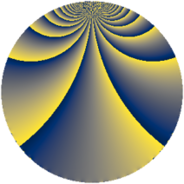# Properties

 Label 2001.2.kLevel $2001$ Weight $2$ Character orbit 2001.k Rep. character $\chi_{2001}(505,\cdot)$ Character field $\Q(\zeta_{4})$ Dimension $240$ Sturm bound $480$

# Related objects

## Defining parameters

 Level: $$N$$ $$=$$ $$2001 = 3 \cdot 23 \cdot 29$$ Weight: $$k$$ $$=$$ $$2$$ Character orbit: $$[\chi]$$ $$=$$ 2001.k (of order $$4$$ and degree $$2$$) Character conductor: $$\operatorname{cond}(\chi)$$ $$=$$ $$667$$ Character field: $$\Q(i)$$ Sturm bound: $$480$$

## Dimensions

The following table gives the dimensions of various subspaces of $$M_{2}(2001, [\chi])$$.

Total New Old
Modular forms 488 240 248
Cusp forms 472 240 232
Eisenstein series 16 0 16

## Trace form

 $$240q + 8q^{2} - 8q^{8} + O(q^{10})$$ $$240q + 8q^{2} - 8q^{8} - 224q^{16} - 8q^{18} + 24q^{23} + 208q^{25} - 24q^{26} + 8q^{31} + 32q^{32} - 240q^{36} - 16q^{39} + 8q^{41} - 20q^{46} - 24q^{47} - 224q^{49} + 16q^{50} - 56q^{55} + 24q^{58} + 16q^{59} - 72q^{70} - 8q^{72} + 40q^{73} - 16q^{75} + 56q^{77} - 240q^{81} - 48q^{82} - 40q^{85} - 8q^{87} + 32q^{94} + 16q^{95} - 136q^{98} + O(q^{100})$$

## Decomposition of $$S_{2}^{\mathrm{new}}(2001, [\chi])$$ into newform subspaces

The newforms in this space have not yet been added to the LMFDB.

## Decomposition of $$S_{2}^{\mathrm{old}}(2001, [\chi])$$ into lower level spaces

$$S_{2}^{\mathrm{old}}(2001, [\chi]) \cong$$ $$S_{2}^{\mathrm{new}}(667, [\chi])$$$$^{\oplus 2}$$